# Automata - Nondeterministic automata (NFA)

Nondeterministic finite automata (NFA) is a finite automata that can be in several states at once (several variable)

The inverse of Nondeterministic automata (NFA) is deterministic finite-state automata (DFA) that cannot be in more than one state at any one time.

## Application

Nondeterminism allows to program solutions to problems using a higher-level language.

The nondetemministic finite automaton is then compiled into a deterministic automaton.

Recommended Pages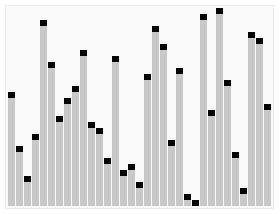Algorithm

An is a (procedure|method) for solving a problem. If there exists an algorithm, the function that performs it is called computable. Study of algorithms dates at least to Euclid and were formalized by...Algorithm - NP Complete (Nondeterministic Polynomial Time Complete)

This is a class of problems that are so difficult that even the best solutions cannot consistently determine their solutions in an efficient way. Specifically, NP Complete problems can only possibly...Automata - Automaton Library

This page lists state machine libraries. All regular expression library implements an automaton. We list a couple of example. : Finite-state automaton for regular...Automata - Deterministic finite-state automata (DFA)

A Deterministic finite-state automata (DFA) is a finite automaton that cannot be in more than one state at any one time. The term deterministic refers to the fact that on each input there is one and only...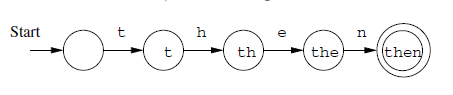Automata - Finite Automata

A finite automaton is an automaton that has a set of states and its control moves from state to state in response to external inputs. It has a start and an end state and there are only a finite number...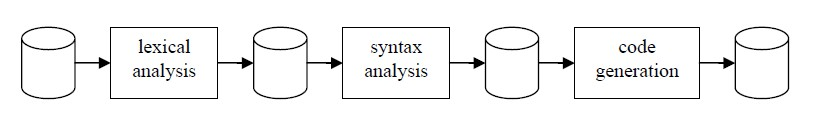Grammar - Hierarchy of grammar (Chomsky)

In the formal languages (of computer science and linguistics), the Chomsky hierarchy is a hierarchy of formal grammars described by Noam Chomsky in 1956. The hierarchy describes the relations between:...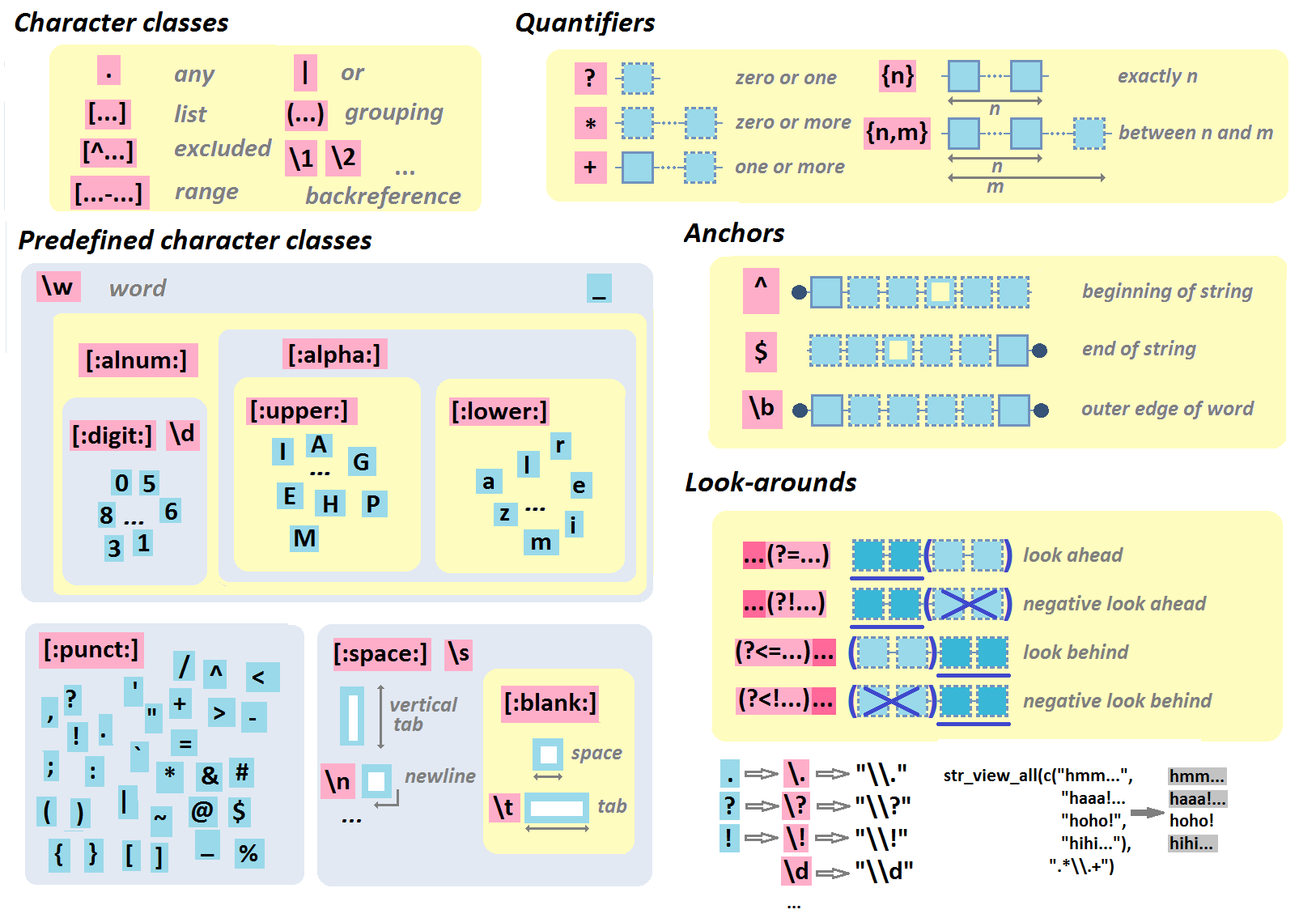Multilingual Regular Expression Syntax (Pattern)

Regular expression are Expression that defines a pattern in text. This is therefore a language that permits to define structure of a text. They are a mathematically-defined concept, invented by Stephen...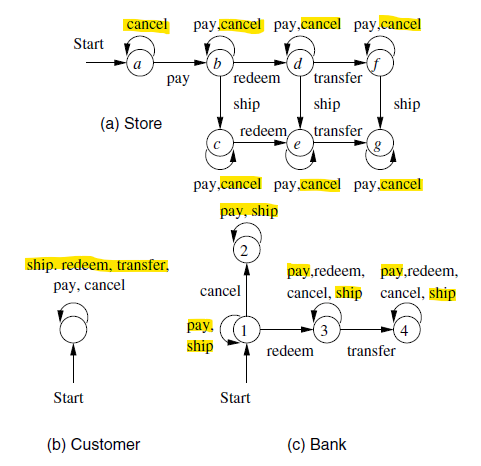Process - LifeCycle (Entity State) (Status)

In a process based model, every entity has a lifecycle (Status) that shows a finite number of distinct states. The status shows the state of an entity ie: finite automata See also: Sequence:...Regexp - Perl-compatible regular expressions (PCRE)

Perl-compatible regular expressions (PCRE) is a regular expression engine that is used in most programming language. It's implemented as a nondeterministic automata (NFA) implementation. The specification...